# Double series

(diff) ← Older revision | Latest revision (diff) | Newer revision → (diff)

A series(1)

the terms,of which form a double sequence of numbers. The finite sums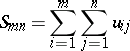are said to be the partial sums of the double series (1) or its rectangular partial sums. They also form a double sequence. If this sequence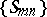has a finite double limit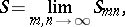(2)

the series (1) is said to be convergent, and the numberis said to be its sum: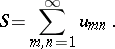If there is no finite limit (2), the series (1) is said to be divergent. Double series have many of the properties of ordinary (single) series. For instance, if the double series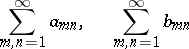converge, then, for any numbersand, the double seriesalso converges and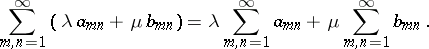If a double series converges, then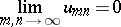(a necessary condition for convergence of the series (1)). For the double series (1) to converge it is necessary and sufficient that for anythere exists a number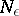such that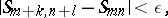provided that,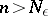, andandare arbitrary non-negative integers. If all terms of the series (1) are non-negative, the sequence of its partial sums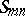always has a finite or an infinite limit, andThe specific properties of a double series are due to the presence of double-indexed terms. If the double series (1) converges and if for all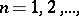the series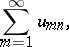converge as well, then the repeated series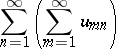also converges, and its sum is equal to the sum of the given series.

A double series is said to converge absolutely if the series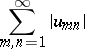converges. If a double series is absolutely convergent, it is also convergent; moreover, any series obtained by rearrangement of its terms also converges, and the sum of any such arbitrary series is the same as the sum of the initial series.

A double series whose terms are functions displays many properties of ordinary series of functions and many concepts are common to both, including the concept of uniform convergence, the Cauchy criterion of uniform convergence of a series or the Weierstrass criterion of uniform convergence. Nevertheless, many theorems valid for ordinary series cannot be directly applied to double series. Thus, the direct analogue of the Abel theorem for power series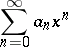does not apply to double power series, i.e. to series of the type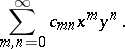(3)

There exist, for example, double series (3) which converge at two points in the plane only: The series (3) with coefficientsconverges only at the two pointsand.

Besides the definition (2) for a double series (1) there also exist other definitions of its convergence and its sum, which are also connected with the double indexation of its terms. For instance, let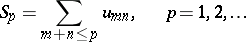(is said to be a triangular partial sum of the double series (1)); the double series (1) will be convergent if the sequenceis convergent; its limit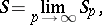is known as the triangular sum of the series (1). If one puts(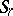is said to be a circular partial sum), then the double series (1) is said to be convergent if the functionof the parameterhas a limit as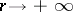, and this limit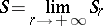is said to be the circular limit of the series (1).

Let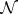denote an arbitrary finite set of index pairsand put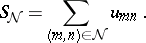Then the numberis said to be the sum of the series (1) if for anythere exists a finite set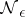of pairs of indices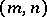such that for any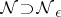the inequality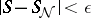is valid. If such a numberexists, the series (1) is convergent.

The definitions of convergence of a series (1) listed above are not mutually equivalent. However, if the terms of the double series are non-negative, convergence in one of the above senses entails convergence in all other senses as well, and the values of the sums of (1) in all cases then coincide. Different summation methods exist for double series.

The concept of a double series can be generalized to series whose terms are not numbers but, for example, elements of a normed linear space.

How to Cite This Entry:
Double series. Encyclopedia of Mathematics. URL: http://encyclopediaofmath.org/index.php?title=Double_series&oldid=12784
This article was adapted from an original article by L.D. Kudryavtsev (originator), which appeared in Encyclopedia of Mathematics - ISBN 1402006098. See original article